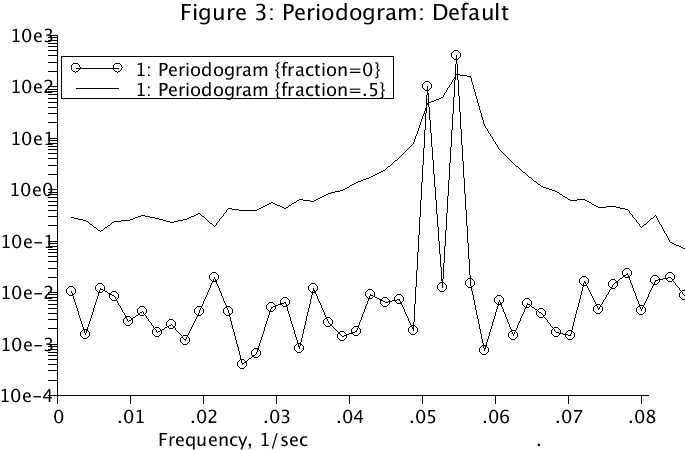# ResolvingFreq

Model number
0355

Spill over of energy into adjacent frequencies is illustrated.

## Description

``` A series is constructed from the following function:

input = 10*cos(2*PI*t*f1)+20*sin(2*PI*t*f2)+randomg()

where f1 = 26/(t.ct*t.delta) and f2 = 28/(t.ct*t.delta).
The periodogram is computed. Then using loops, f1 and f2 are
increased by a small amount:

f1 = 26.571428/(t.ct*t.delta) and f2 = 28.571428/(t.ct*t.delta).

The periodogram now shows leakage of energy from the original
two peaks into adjacent frequencies and the two frequencies
are not resolved. This is seen on plotpage Periodogram by
running Loops. A partial solution is to sample for a longer
time to resolve the frequencies, but this may not resolve
the leakage problem.```This is a periodogram of an input function with frequencies which are exactly matched by the Fourier transform frequencies (solid line with circles). Then an input function is generated with slightly different frequencies and the energy spills over into adjacent frequencies and the two modified frequencies are not resolved.

## Equations

None.

The equations for this model may be viewed by running the JSim model applet and clicking on the Source tab at the bottom left of JSim's Run Time graphical user interface. The equations are written in JSim's Mathematical Modeling Language (MML). See the Introduction to MML and the MML Reference Manual. Additional documentation for MML can be found by using the search option at the Physiome home page.

References

None

Key terms
resolution
Fourier
forward
backward
transform
periodogram
time series
frequency